The VARMAX Procedure

Vector Autoregressive Process

Subsections:

Let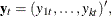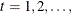denote a k-dimensional time series vector of random variables of interest. The pth-order VAR process is written as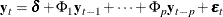where the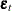is a vector white noise process with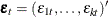such that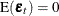,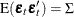, and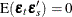for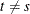;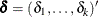is a constant vector and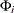is a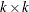matrix.

Analyzing and modeling the series jointly enables you to understand the dynamic relationships over time among the series and to improve the accuracy of forecasts for individual series by using the additional information available from the related series and their forecasts.

Example of Vector Autoregressive Model

Consider the first-order stationary bivariate vector autoregressive model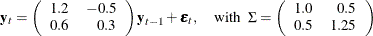The following IML procedure statements simulate a bivariate vector time series from this model to provide test data for the VARMAX procedure:

proc iml;
sig = {1.0  0.5, 0.5 1.25};
phi = {1.2 -0.5, 0.6 0.3};
/* simulate the vector time series */
call varmasim(y,phi) sigma = sig n = 100 seed = 34657;
cn = {'y1' 'y2'};
create simul1 from y[colname=cn];
append from y;
quit;


The following statements plot the simulated vector time series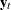shown in Figure 35.1:

data simul1;
set simul1;
date = intnx( 'year', '01jan1900'd, _n_-1 );
format date year4.;
run;

ods graphics on;
proc timeseries data=simul1 vectorplot=series;
id date interval=year;
var y1 y2;
run;


Figure 35.1: Plot of Generated Data Process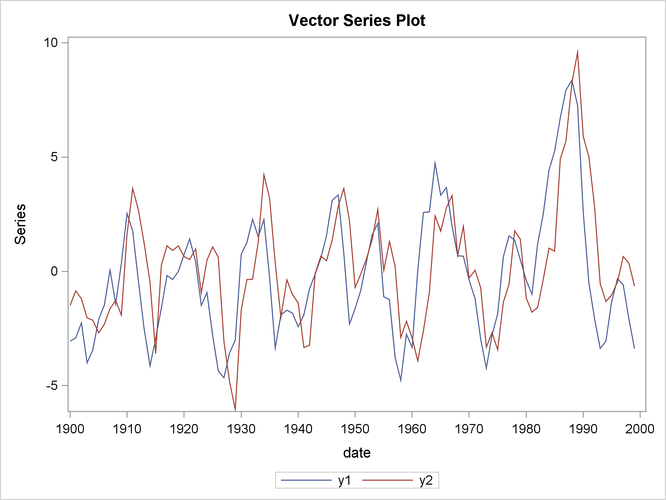The following statements fit a VAR(1) model to the simulated data. First, you specify the input data set in the PROC VARMAX statement. Then, you use the MODEL statement to designate the dependent variables,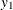and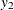. To estimate a VAR model with mean zero, you specify the order of the autoregressive model with the P= option and the NOINT option. The MODEL statement fits the model to the data and prints parameter estimates and their significance. The PRINT=ESTIMATES option prints the matrix form of parameter estimates, and the PRINT=DIAGNOSE option prints various diagnostic tests. The LAGMAX=3 option is used to print the output for the residual diagnostic checks.

To output the forecasts to a data set, you specify the OUTPUT statement with the OUT= option. If you want to forecast five steps ahead, you use the LEAD=5 option. The ID statement specifies the yearly interval between observations and provides the Time column in the forecast output.

The VARMAX procedure output is shown in Figure 35.2 through Figure 35.10.

/*--- Vector Autoregressive Model ---*/

proc varmax data=simul1;
id date interval=year;
model y1 y2 / p=1 noint lagmax=3
print=(estimates diagnose);
run;


Figure 35.2: Descriptive Statistics

The VARMAX Procedure

 Number of Observations 100 0

Simple Summary Statistics
Variable Type N Mean Standard
Deviation
Min Max
y1 Dependent 100 -0.21653 2.78210 -4.75826 8.37032
y2 Dependent 100 0.16905 2.58184 -6.04718 9.58487

The VARMAX procedure first displays descriptive statistics. The Type column specifies that the variables are dependent variables. The column N stands for the number of nonmissing observations.

Figure 35.3 shows the type and the estimation method of the fitted model for the simulated data. It also shows the AR coefficient matrix in terms of lag 1, the parameter estimates, and their significance, which can indicate how well the model fits the data.

The second table schematically represents the parameter estimates and allows for easy verification of their significance in matrix form.

In the last table, the first column gives the left-hand-side variable of the equation; the second column is the parameter name AR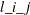, which indicates the (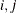)th element of the lag l autoregressive coefficient; the last column is the regressor that corresponds to the displayed parameter.

Figure 35.3: Model Type and Parameter Estimates

The VARMAX Procedure

Type of Model VAR(1) Least Squares Estimation

AR
Lag Variable y1 y2
1 y1 1.15977 -0.51058
y2 0.54634 0.38499

Schematic
Representation
Variable/Lag AR1
y1 +-
y2 ++

Model Parameter Estimates
Equation Parameter Estimate Standard
Error
t Value Pr > |t| Variable
y1 AR1_1_1 1.15977 0.05508 21.06 0.0001 y1(t-1)
AR1_1_2 -0.51058 0.05898 -8.66 0.0001 y2(t-1)
y2 AR1_2_1 0.54634 0.05779 9.45 0.0001 y1(t-1)
AR1_2_2 0.38499 0.06188 6.22 0.0001 y2(t-1)

The fitted VAR(1) model with estimated standard errors in parentheses is given as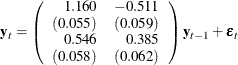Clearly, all parameter estimates in the coefficient matrix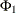are significant.

The model can also be written as two univariate regression equations.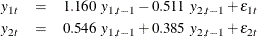The table in Figure 35.4 shows the innovation covariance matrix estimates and the various information criteria results. The smaller value of information criteria fits the data better when it is compared to other models. The variable names in the covariance matrix are printed for convenience;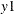means the innovation for, and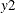means the innovation for.

Figure 35.4: Innovation Covariance Estimates and Information Criteria

Covariances of Innovations
Variable y1 y2
y1 1.28875 0.39751
y2 0.39751 1.41839

Information Criteria
AICC 0.554443
HQC 0.595201
AIC 0.552777
SBC 0.65763
FPEC 1.738092

Figure 35.5 shows the cross covariances of the residuals. The values of the lag zero are slightly different from Figure 35.4 due to the different degrees of freedom.

Figure 35.5: Multivariate Diagnostic Checks

Cross Covariances of Residuals
Lag Variable y1 y2
0 y1 1.26271 0.38948
y2 0.38948 1.38974
1 y1 0.03121 0.05675
y2 -0.04646 -0.05398
2 y1 0.08134 0.10599
y2 0.03482 -0.01549
3 y1 0.01644 0.11734
y2 0.00609 0.11414

Figure 35.6 and Figure 35.7 show tests for white noise residuals. The output shows that you cannot reject the null hypothesis that the residuals are uncorrelated.

Figure 35.6: Multivariate Diagnostic Checks Continued

Cross Correlations of Residuals
Lag Variable y1 y2
0 y1 1.00000 0.29401
y2 0.29401 1.00000
1 y1 0.02472 0.04284
y2 -0.03507 -0.03884
2 y1 0.06442 0.08001
y2 0.02628 -0.01115
3 y1 0.01302 0.08858
y2 0.00460 0.08213

Schematic Representation
of Cross Correlations
of Residuals
Variable/Lag 0 1 2 3
y1 ++ .. .. ..
y2 ++ .. .. ..

Figure 35.7: Multivariate Diagnostic Checks Continued

Portmanteau Test for Cross Correlations
of Residuals
Up To Lag DF Chi-Square Pr > ChiSq
2 4 1.58 0.8124
3 8 2.78 0.9473

The VARMAX procedure provides diagnostic checks for the univariate form of the equations. The table in Figure 35.8 describes how well each univariate equation fits the data. From two univariate regression equations in Figure 35.3, the values of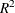in the second column are 0.84 and 0.80 for each equation. The standard deviations in the third column are the square roots of the diagonal elements of the covariance matrix from Figure 35.4. The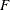statistics are in the fourth column for hypotheses to test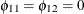and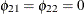, respectively, where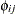is the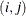th element of the matrix. The last column shows the p-values of thestatistics. The results show that each univariate model is significant.

Figure 35.8: Univariate Diagnostic Checks

Univariate Model ANOVA Diagnostics
Variable R-Square Standard
Deviation
F Value Pr > F
y1 0.8351 1.13523 491.25 <.0001
y2 0.7906 1.19096 366.29 <.0001

The check for white noise residuals in terms of the univariate equation is shown in Figure 35.9. This output contains information that indicates whether the residuals are correlated and heteroscedastic. In the first table, the second column contains the Durbin-Watson test statistics to test the null hypothesis that the residuals are uncorrelated. The third and fourth columns show the Jarque-Bera normality test statistics and their p-values to test the null hypothesis that the residuals have normality. The last two columns showstatistics and their p-values for ARCH(1) disturbances to test the null hypothesis that the residuals have equal covariances. The second table includesstatistics and their p-values for AR(1), AR(1,2), AR(1,2,3) and AR(1,2,3,4) models of residuals to test the null hypothesis that the residuals are uncorrelated.

Figure 35.9: Univariate Diagnostic Checks Continued

Univariate Model White Noise Diagnostics
Variable Durbin
Watson
Normality ARCH
Chi-Square Pr > ChiSq F Value Pr > F
y1 1.94534 3.56 0.1686 0.13 0.7199
y2 2.06276 5.42 0.0667 2.10 0.1503

Univariate Model AR Diagnostics
Variable AR1 AR2 AR3 AR4
F Value Pr > F F Value Pr > F F Value Pr > F F Value Pr > F
y1 0.02 0.8980 0.14 0.8662 0.09 0.9629 0.82 0.5164
y2 0.52 0.4709 0.41 0.6650 0.32 0.8136 0.32 0.8664

The table in Figure 35.10 gives forecasts, their prediction errors, and 95% confidence limits. See the section Forecasting for details.

Figure 35.10: Forecasts

Forecasts
Variable Obs Time Forecast Standard
Error
95% Confidence Limits
y1 101 2000 -3.59212 1.13523 -5.81713 -1.36711
102 2001 -3.09448 1.70915 -6.44435 0.25539
103 2002 -2.17433 2.14472 -6.37792 2.02925
104 2003 -1.11395 2.43166 -5.87992 3.65203
105 2004 -0.14342 2.58740 -5.21463 4.92779
y2 101 2000 -2.09873 1.19096 -4.43298 0.23551
102 2001 -2.77050 1.47666 -5.66469 0.12369
103 2002 -2.75724 1.74212 -6.17173 0.65725
104 2003 -2.24943 2.01925 -6.20709 1.70823
105 2004 -1.47460 2.25169 -5.88782 2.93863